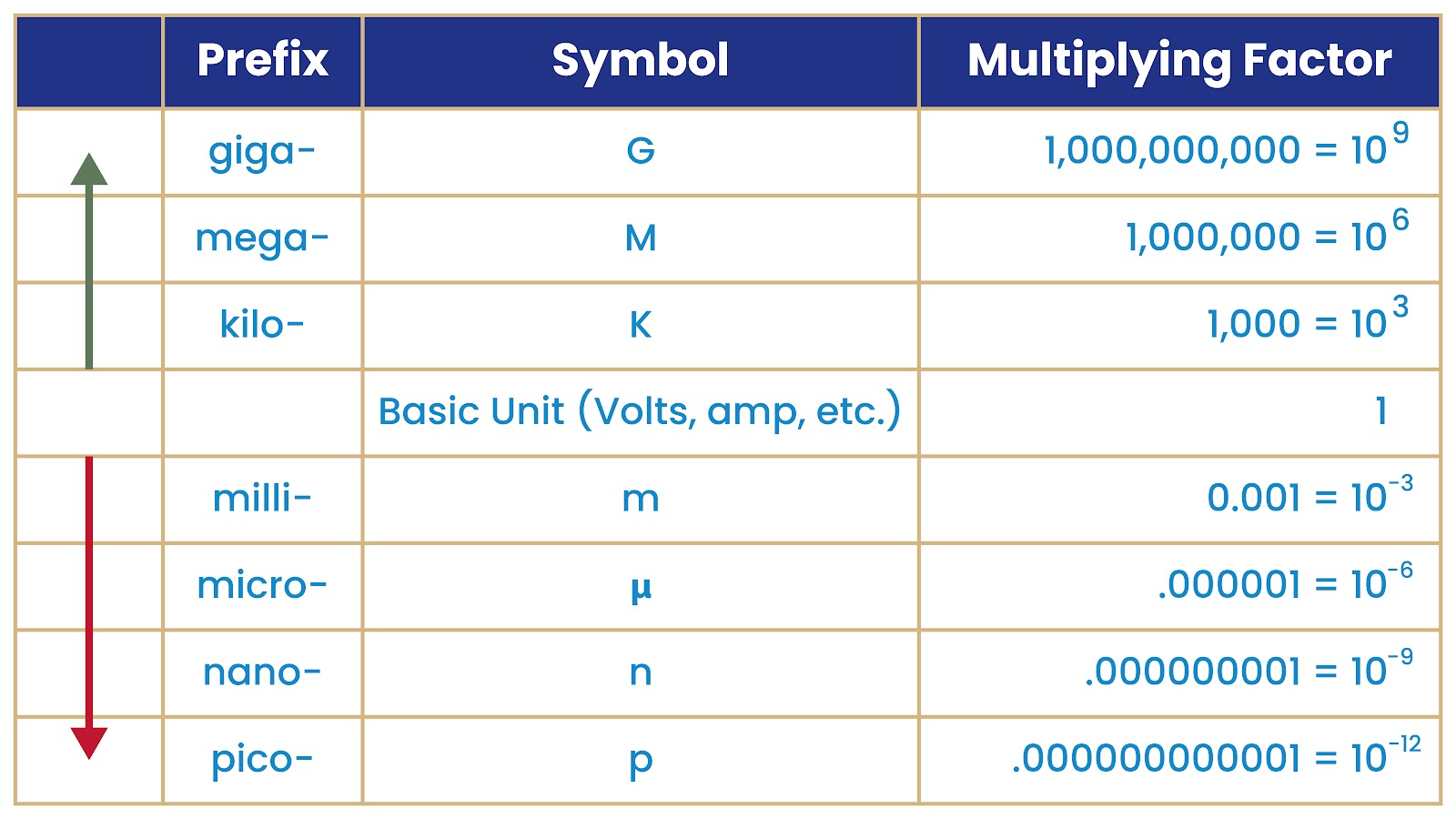<< index

by disinfoniacs #69 & #1

## units

>>>>

### tldr

• Giga (G): 1,000,000,000 (10^9) times the base unit
• Mega (M): 1,000,000 (10^6) times the base unit
• Kilo (k): 1,000 (10^3) times the base unit
• Milli (m): 0.001 (10^-3) times the base unit
• Micro (μ): 0.000001 (10^-6) times the base unit
• Nano (n): 0.000000001 (10^-9) times the base unit
• Pico (p): 0.000000000001 (10^-12) times the base unit

## in depthThe metric prefixes chart is useful for understanding the different magnitudes of measurement units used in science, engineering, and other fields that deal with quantities that span several orders of magnitude. These prefixes allow us to express very large or very small values in a concise and easy-to-read format.

For example, it would be difficult and cumbersome to write out or say the value of 0.00000000123 seconds, but much easier to write or say "1.23 nanoseconds". Similarly, it would be cumbersome to write or say the value of 1,000,000,000 watts, but much easier to write or say "1 gigawatt".

This chart can be particularly useful when working with electronic components or circuits, as they often involve very small or very large quantities of electrical charge, voltage, resistance, and power. The metric prefixes help us express these values in a way that is easy to read, write, and remember.

Each prefix represents a multiplying factor of 10 raised to a certain power, based on the prefix's name. For example, "kilo" means "thousand", so the prefix "k" represents a multiplying factor of 1000.

To use the chart, simply find the appropriate prefix for the unit you're working with, and use the corresponding multiplying factor to convert to the desired unit. For example, if you have a measurement of 500 millimeters and you want to convert it to meters, you would use the milli prefix, which has a multiplying factor of 0.001. So:

500 millimeters = 500 x 0.001 meters = 0.5 meters

---

<< index